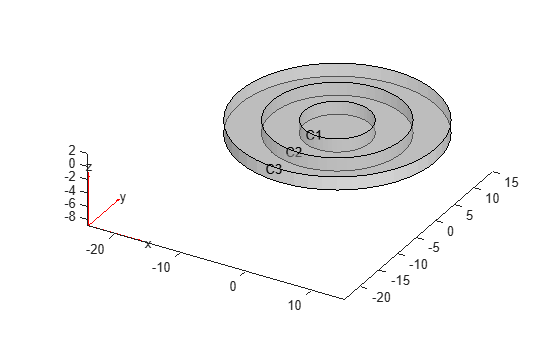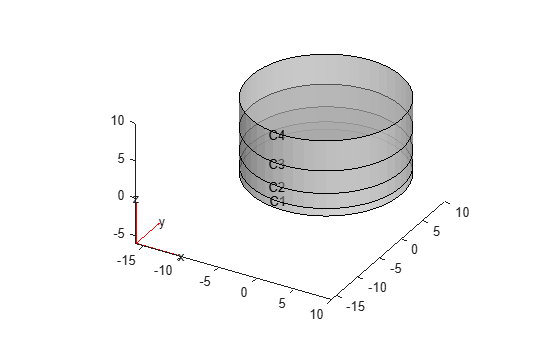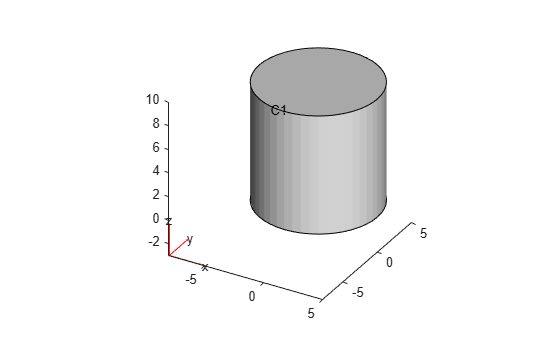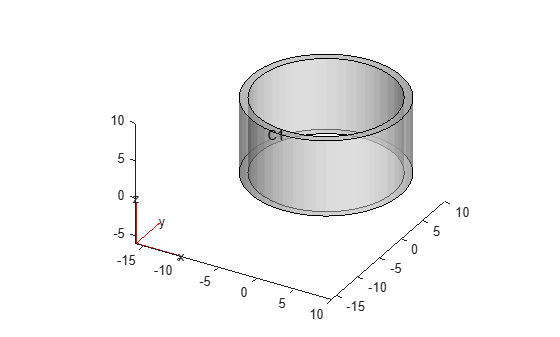# multicylinder

Create geometry formed by several cylindrical cells

## Syntax

``gm = multicylinder(R,H)``
``gm = multicylinder(R,H,Name,Value)``

## Description

example

````gm = multicylinder(R,H)` creates a geometry by combining several cylindrical cells.When creating each cylinder, `multicylinder` uses the following coordinate system.```

example

````gm = multicylinder(R,H,Name,Value)` creates a multi-cylinder geometry using one or more `Name,Value` pair arguments.```

## Examples

collapse all

Create a geometry that consists of three nested cylinders of the same height and include this geometry in a PDE model.

Create the geometry by using the `multicylinder` function. The resulting geometry consists of three cells.

`gm = multicylinder([5 10 15],2)`
```gm = DiscreteGeometry with properties: NumCells: 3 NumFaces: 9 NumEdges: 6 NumVertices: 6 Vertices: [6x3 double] ```

Create a PDE model.

`model = createpde`
```model = PDEModel with properties: PDESystemSize: 1 IsTimeDependent: 0 Geometry: [] EquationCoefficients: [] BoundaryConditions: [] InitialConditions: [] Mesh: [] SolverOptions: [1x1 pde.PDESolverOptions] ```

Include the geometry in the model.

`model.Geometry = gm`
```model = PDEModel with properties: PDESystemSize: 1 IsTimeDependent: 0 Geometry: [1x1 DiscreteGeometry] EquationCoefficients: [] BoundaryConditions: [] InitialConditions: [] Mesh: [] SolverOptions: [1x1 pde.PDESolverOptions] ```

Plot the geometry.

`pdegplot(model,'CellLabels','on','FaceAlpha',0.5)`Create a geometry that consists of three stacked cylinders and include this geometry in a PDE model.

Create the geometry by using the `multicylinder` function with the `ZOffset` argument. The resulting geometry consists of four cells stacked on top of each other.

`gm = multicylinder(10,[1 2 3 4],'ZOffset',[0 1 3 6])`
```gm = DiscreteGeometry with properties: NumCells: 4 NumFaces: 9 NumEdges: 5 NumVertices: 5 Vertices: [5x3 double] ```

Create a PDE model.

`model = createpde`
```model = PDEModel with properties: PDESystemSize: 1 IsTimeDependent: 0 Geometry: [] EquationCoefficients: [] BoundaryConditions: [] InitialConditions: [] Mesh: [] SolverOptions: [1x1 pde.PDESolverOptions] ```

Include the geometry in the model.

`model.Geometry = gm`
```model = PDEModel with properties: PDESystemSize: 1 IsTimeDependent: 0 Geometry: [1x1 DiscreteGeometry] EquationCoefficients: [] BoundaryConditions: [] InitialConditions: [] Mesh: [] SolverOptions: [1x1 pde.PDESolverOptions] ```

Plot the geometry.

`pdegplot(model,'CellLabels','on','FaceAlpha',0.5)`Create a geometry that consists of a single cylinder and include this geometry in a PDE model.

Use the `multicylinder` function to create a single cylinder. The resulting geometry consists of one cell.

`gm = multicylinder(5,10)`
```gm = DiscreteGeometry with properties: NumCells: 1 NumFaces: 3 NumEdges: 2 NumVertices: 2 Vertices: [2x3 double] ```

Create a PDE model.

`model = createpde`
```model = PDEModel with properties: PDESystemSize: 1 IsTimeDependent: 0 Geometry: [] EquationCoefficients: [] BoundaryConditions: [] InitialConditions: [] Mesh: [] SolverOptions: [1x1 pde.PDESolverOptions] ```

Include the geometry in the model.

`model.Geometry = gm`
```model = PDEModel with properties: PDESystemSize: 1 IsTimeDependent: 0 Geometry: [1x1 DiscreteGeometry] EquationCoefficients: [] BoundaryConditions: [] InitialConditions: [] Mesh: [] SolverOptions: [1x1 pde.PDESolverOptions] ```

Plot the geometry.

`pdegplot(model,'CellLabels','on')`Create a hollow cylinder and include it as a geometry in a PDE model.

Create a hollow cylinder by using the `multicylinder` function with the `Void` argument. The resulting geometry consists of one cell.

`gm = multicylinder([9 10],10,'Void',[true,false])`
```gm = DiscreteGeometry with properties: NumCells: 1 NumFaces: 4 NumEdges: 4 NumVertices: 4 Vertices: [4x3 double] ```

Create a PDE model.

`model = createpde`
```model = PDEModel with properties: PDESystemSize: 1 IsTimeDependent: 0 Geometry: [] EquationCoefficients: [] BoundaryConditions: [] InitialConditions: [] Mesh: [] SolverOptions: [1x1 pde.PDESolverOptions] ```

Include the geometry in the model.

`model.Geometry = gm`
```model = PDEModel with properties: PDESystemSize: 1 IsTimeDependent: 0 Geometry: [1x1 DiscreteGeometry] EquationCoefficients: [] BoundaryConditions: [] InitialConditions: [] Mesh: [] SolverOptions: [1x1 pde.PDESolverOptions] ```

Plot the geometry.

`pdegplot(model,'CellLabels','on','FaceAlpha',0.5)`## Input Arguments

collapse all

Cell radius, specified as a positive real number or a vector of positive real numbers. If `R` is a vector, then `R(i)` specifies the radius of the `i`th cell.

Radius `R` and height `H` can be scalars or vectors of the same length. For a combination of scalar and vector inputs, `multicylinder` replicates the scalar arguments into vectors of the same length.

Note

Either radius or height must be the same for all cells in the geometry.

Example: `gm = multicylinder([1 2 3],1)`

Cell height, specified as a positive real number or a vector of positive real numbers. If `H` is a vector, then `H(i)` specifies the height of the `i`th cell.

Radius `R` and height `H` can be scalars or vectors of the same length. For a combination of scalar and vector inputs, `multicylinder` replicates the scalar arguments into vectors of the same length.

Note

Either radius or height must be the same for all cells in the geometry.

Example: `gm = multicylinder(1,[1 2 3],'Zoffset',[0 1 3])`

### Name-Value Arguments

Specify optional comma-separated pairs of `Name,Value` arguments. `Name` is the argument name and `Value` is the corresponding value. `Name` must appear inside quotes. You can specify several name and value pair arguments in any order as `Name1,Value1,...,NameN,ValueN`.

Example: `gm = multicylinder([1 2],1,'Void',[true,false])`

Z-offset for each cell, specified as a vector of real numbers. `ZOffset(i)` specifies the Z-offset of the `i`th cell. This vector must have the same length as the radius vector `R` or height vector `H`.

Note

The `ZOffset` argument is valid only if the radius is the same for all cells in the geometry.

Example: ```gm = multicylinder(20,[10 10],'ZOffset',[0 10])```

Data Types: `double`

Empty cell indicator, specified as a vector of logical `true` or `false` values. This vector must have the same length as the radius vector `R` or the height vector `H`.

The value `true` corresponds to an empty cell. By default, `multicylinder` assumes that all cells are not empty.

Example: `gm = multicylinder([1 2],1,'Void',[true,false])`

Data Types: `double`

## Output Arguments

collapse all

Geometry object, returned as a DiscreteGeometry Properties object.

Tip

A cylinder has one cell, three faces, and two edges. Also, since every edge has a start and an end vertex, a cylinder has vertices. Both edges are circles, their start and end vertices coincide. Thus, a cylinder has two vertices - one for each edge.

## Limitations

• `multicylinder` lets you create only geometries consisting of stacked or nested cylinders. For nested cylinders, the height must be the same for all cells in the geometry. For stacked cylinders, the radius must be the same for all cells in the geometry. Use the `ZOffset` argument to stack the cells on top of each over without overlapping them.

• `multicylinder` does not let you create nested cylinders of the same radius. The call `multicylinder(r,[h1,h2,...])` is not supported.